The Normal Distribution

# 30 The Standard Normal Distribution

The standard normal distribution is a normal distribution of standardized values called z-scores. A z-score is measured in units of the standard deviation.

The mean for the standard normal distribution is zero, and the standard deviation is one. What this does is dramatically simplify the mathematical calculation of probabilities. Take a moment and substitute zero and one in the appropriate places in the above formula and you can see that the equation collapses into one that can be much more easily solved using integral calculus. The transformation z =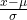produces the distribution Z ~ N(0, 1). The value x in the given equation comes from a known normal distribution with known mean μ and known standard deviation σ. The z-score tells how many standard deviations a particular x is away from the mean.

### Z-Scores

If X is a normally distributed random variable and X ~ N(μ, σ), then the z-score for a particular x is: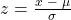The z-score tells you how many standard deviations the value x is above (to the right of) or below (to the left of) the mean, μ. Values of x that are larger than the mean have positive z-scores, and values of x that are smaller than the mean have negative z-scores. If x equals the mean, then x has a z-score of zero.

Suppose X ~ N(5, 6). This says that X is a normally distributed random variable with mean μ = 5 and standard deviation σ = 6. Suppose x = 17. Then: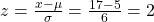This means that x = 17 is two standard deviations (2σ) above or to the right of the mean μ = 5.

Now suppose x = 1. Then: z ==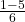= –0.67 (rounded to two decimal places)

This means that x = 1 is 0.67 standard deviations (–0.67σ) below or to the left of the mean μ = 5.

The Empirical RuleIf X is a random variable and has a normal distribution with mean µ and standard deviation σ, then the Empirical Rule states the following:

• About 68% of the x values lie between –1σ and +1σ of the mean µ (within one standard deviation of the mean).
• About 95% of the x values lie between –2σ and +2σ of the mean µ (within two standard deviations of the mean).
• About 99.7% of the x values lie between –3σ and +3σ of the mean µ (within three standard deviations of the mean). Notice that almost all the x values lie within three standard deviations of the mean.
• The z-scores for +1σ and –1σ are +1 and –1, respectively.
• The z-scores for +2σ and –2σ are +2 and –2, respectively.
• The z-scores for +3σ and –3σ are +3 and –3 respectively.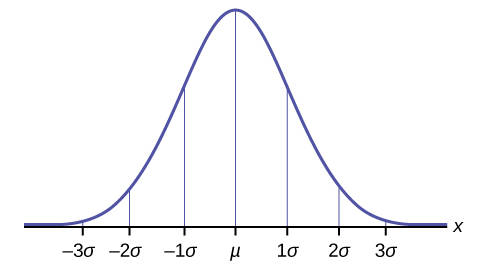Suppose x has a normal distribution with mean 50 and standard deviation 6.

• About 68% of the x values lie within one standard deviation of the mean. Therefore, about 68% of the x values lie between –1σ = (–1)(6) = –6 and 1σ = (1)(6) = 6 of the mean 50. The values 50 – 6 = 44 and 50 + 6 = 56 are within one standard deviation from the mean 50. The z-scores are –1 and +1 for 44 and 56, respectively.
• About 95% of the x values lie within two standard deviations of the mean. Therefore, about 95% of the x values lie between –2σ = (–2)(6) = –12 and 2σ = (2)(6) = 12. The values 50 – 12 = 38 and 50 + 12 = 62 are within two standard deviations from the mean 50. The z-scores are –2 and +2 for 38 and 62, respectively.
• About 99.7% of the x values lie within three standard deviations of the mean. Therefore, about 95% of the x values lie between –3σ = (–3)(6) = –18 and 3σ = (3)(6) = 18 of the mean 50. The values 50 – 18 = 32 and 50 + 18 = 68 are within three standard deviations from the mean 50. The z-scores are –3 and +3 for 32 and 68, respectively.

### References

“Blood Pressure of Males and Females.” StatCruch, 2013. Available online at http://www.statcrunch.com/5.0/viewreport.php?reportid=11960 (accessed May 14, 2013).

“The Use of Epidemiological Tools in Conflict-affected populations: Open-access educational resources for policy-makers: Calculation of z-scores.” London School of Hygiene and Tropical Medicine, 2009. Available online at http://conflict.lshtm.ac.uk/page_125.htm (accessed May 14, 2013).

“2012 College-Bound Seniors Total Group Profile Report.” CollegeBoard, 2012. Available online at http://media.collegeboard.com/digitalServices/pdf/research/TotalGroup-2012.pdf (accessed May 14, 2013).

“Digest of Education Statistics: ACT score average and standard deviations by sex and race/ethnicity and percentage of ACT test takers, by selected composite score ranges and planned fields of study: Selected years, 1995 through 2009.” National Center for Education Statistics. Available online at http://nces.ed.gov/programs/digest/d09/tables/dt09_147.asp (accessed May 14, 2013).

Data from the San Jose Mercury News.

Data from The World Almanac and Book of Facts.

“List of stadiums by capacity.” Wikipedia. Available online at https://en.wikipedia.org/wiki/List_of_stadiums_by_capacity (accessed May 14, 2013).

Data from the National Basketball Association. Available online at www.nba.com (accessed May 14, 2013).

### Chapter Review

A z-score is a standardized value. Its distribution is the standard normal, Z ~ N(0, 1). The mean of the z-scores is zero and the standard deviation is one. If z is the z-score for a value x from the normal distribution N(µ, σ) then z tells you how many standard deviations x is above (greater than) or below (less than) µ.

### Formula Review

Z ~ N(0, 1)

z = a standardized value (z-score)

mean = 0; standard deviation = 1

To find the kth percentile of X when the z-scores is known:
k = μ + (z)σ

z-score: z =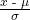or z =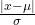Z = the random variable for z-scores

Z ~ N(0, 1)

A bottle of water contains 12.05 fluid ounces with a standard deviation of 0.01 ounces. Define the random variable X in words. X = ____________.

ounces of water in a bottle

A normal distribution has a mean of 61 and a standard deviation of 15. What is the median?

<!– <solution id=”fs-idm63161360″> 61 –>

X ~ N(1, 2)

σ = _______

2

A company manufactures rubber balls. The mean diameter of a ball is 12 cm with a standard deviation of 0.2 cm. Define the random variable X in words. X = ______________.

<!– <solution id=”fs-idp15866016″> diameter of a rubber ball –>

X ~ N(–4, 1)

What is the median?

–4

X ~ N(3, 5)

σ = _______

<!– <solution id=”fs-idm120454704″> 5 –>

X ~ N(–2, 1)

μ = _______

–2

What does a z-score measure?

<!– <solution id=”fs-idm57247936″> The number of standard deviations a value is from the mean. –>

What does standardizing a normal distribution do to the mean?

The mean becomes zero.

Is X ~ N(0, 1) a standardized normal distribution? Why or why not?

<!– <solution id=”fs-idp48272224″> Yes because the mean is zero, and the standard deviation is one. –>

What is the z-score of x = 12, if it is two standard deviations to the right of the mean?

z = 2

What is the z-score of x = 9, if it is 1.5 standard deviations to the left of the mean?

<!– <solution id=”fs-idp6573504″> z = –1.5 –>

What is the z-score of x = –2, if it is 2.78 standard deviations to the right of the mean?

z = 2.78

What is the z-score of x = 7, if it is 0.133 standard deviations to the left of the mean?

<!– <solution id=”fs-idp38051616″> z = –0.133 –>

Suppose X ~ N(2, 6). What value of x has a z-score of three?

x = 20

Suppose X ~ N(8, 1). What value of x has a z-score of –2.25?

<!– <solution id=”fs-idp19875360″> x = 5.75 –>

Suppose X ~ N(9, 5). What value of x has a z-score of –0.5?

x = 6.5

Suppose X ~ N(2, 3). What value of x has a z-score of –0.67?

<!– <solution id=”fs-idp31923120″> x = –0.01 –>

Suppose X ~ N(4, 2). What value of x is 1.5 standard deviations to the left of the mean?

x = 1

Suppose X ~ N(4, 2). What value of x is two standard deviations to the right of the mean?

<!– <solution id=”fs-idm47755696″> x = 8 –>

Suppose X ~ N(8, 9). What value of x is 0.67 standard deviations to the left of the mean?

x = 1.97

Suppose X ~ N(–1, 2). What is the z-score of x = 2?

<!– <solution id=”fs-idm119642576″> z = 1.5 –>

Suppose X ~ N(12, 6). What is the z-score of x = 2?

z = –1.67

Suppose X ~ N(9, 3). What is the z-score of x = 9?

<!– <solution id=”fs-idm56843792″> z = 0 –>

Suppose a normal distribution has a mean of six and a standard deviation of 1.5. What is the z-score of x = 5.5?

z ≈ –0.33

In a normal distribution, x = 5 and z = –1.25. This tells you that x = 5 is ____ standard deviations to the ____ (right or left) of the mean.

<!– <solution id=”fs-idm62539280″> 1.25, left –>

In a normal distribution, x = 3 and z = 0.67. This tells you that x = 3 is ____ standard deviations to the ____ (right or left) of the mean.

0.67, right

In a normal distribution, x = –2 and z = 6. This tells you that x = –2 is ____ standard deviations to the ____ (right or left) of the mean.

<!– <solution id=”fs-idm102069296″> six, right –>

In a normal distribution, x = –5 and z = –3.14. This tells you that x = –5 is ____ standard deviations to the ____ (right or left) of the mean.

3.14, left

In a normal distribution, x = 6 and z = –1.7. This tells you that x = 6 is ____ standard deviations to the ____ (right or left) of the mean.

<!– <solution id=”fs-idm98531584″> 1.7, left –>

About what percent of x values from a normal distribution lie within one standard deviation (left and right) of the mean of that distribution?

About what percent of the x values from a normal distribution lie within two standard deviations (left and right) of the mean of that distribution?

<!– <solution id=”fs-idm47813520″> about 95.45% –>

About what percent of x values lie between the second and third standard deviations (both sides)?

Suppose X ~ N(15, 3). Between what x values does 68.27% of the data lie? The range of x values is centered at the mean of the distribution (i.e., 15).

<!– <solution id=”fs-idm33513040″> between 12 and 18 –>

Suppose X ~ N(–3, 1). Between what x values does 95.45% of the data lie? The range of x values is centered at the mean of the distribution(i.e., –3).

between –5 and –1

Suppose X ~ N(–3, 1). Between what x values does 34.14% of the data lie?

<!– <solution id=”fs-idm68579024″> between –4 and –3 or between –3 and –2 –>

About what percent of x values lie between the mean and three standard deviations?

About what percent of x values lie between the mean and one standard deviation?

<!– <solution id=”fs-idp3556992″> about 34.14% –>

About what percent of x values lie between the first and second standard deviations from the mean (both sides)?

About what percent of x values lie betwween the first and third standard deviations(both sides)?

<!– <solution id=”fs-idp56159920″> about 34.46% –>

Use the following information to answer the next two exercises: The life of Sunshine CD players is normally distributed with mean of 4.1 years and a standard deviation of 1.3 years. A CD player is guaranteed for three years. We are interested in the length of time a CD player lasts.

Define the random variable X in words. X = _______________.

The lifetime of a Sunshine CD player measured in years.

X ~ _____(_____,_____)

<!– <solution id=”fs-idp89883200″> X ~ N(4.1, 1.3) –>

### Homework

Use the following information to answer the next two exercises: The patient recovery time from a particular surgical procedure is normally distributed with a mean of 5.3 days and a standard deviation of 2.1 days.

What is the median recovery time?

1. 2.7
2. 5.3
3. 7.4
4. 2.1

<!– <solution id=”fs-idm68359392″> b –>

What is the z-score for a patient who takes ten days to recover?

1. 1.5
2. 0.2
3. 2.2
4. 7.3

c

The length of time to find it takes to find a parking space at 9 A.M. follows a normal distribution with a mean of five minutes and a standard deviation of two minutes. If the mean is significantly greater than the standard deviation, which of the following statements is true?

1. The data cannot follow the uniform distribution.
2. The data cannot follow the exponential distribution..
3. The data cannot follow the normal distribution.
1. I only
2. II only
3. III only
4. I, II, and III

<!– <solution id=”fs-idp16013392″> b –>

The heights of the 430 National Basketball Association players were listed on team rosters at the start of the 2005–2006 season. The heights of basketball players have an approximate normal distribution with mean, µ = 79 inches and a standard deviation, σ = 3.89 inches. For each of the following heights, calculate the z-score and interpret it using complete sentences.

1. 77 inches
2. 85 inches
3. If an NBA player reported his height had a z-score of 3.5, would you believe him? Explain your answer.
1. Use the z-score formula. z = –0.5141. The height of 77 inches is 0.5141 standard deviations below the mean. An NBA player whose height is 77 inches is shorter than average.
2. Use the z-score formula. z = 1.5424. The height 85 inches is 1.5424 standard deviations above the mean. An NBA player whose height is 85 inches is taller than average.
3. Height = 79 + 3.5(3.89) = 92.615 inches, which is taller than 7 feet, 8 inches. There are very few NBA players this tall so the answer is no, not likely.

The systolic blood pressure (given in millimeters) of males has an approximately normal distribution with mean µ = 125 and standard deviation σ = 14. Systolic blood pressure for males follows a normal distribution.

1. Calculate the z-scores for the male systolic blood pressures 100 and 150 millimeters.
2. If a male friend of yours said he thought his systolic blood pressure was 2.5 standard deviations below the mean, but that he believed his blood pressure was between 100 and 150 millimeters, what would you say to him?

<!– <solution id=”eip-774″> Use the z-score formula. 100 – 125 14 ≈ –1.8 and 100 – 125 14 ≈ 1.8 I would tell him that 2.5 standard deviations below the mean would give him a blood pressure reading of 90, which is below the range of 100 to 150. –>

Kyle’s doctor told him that the z-score for his systolic blood pressure is 1.75. Which of the following is the best interpretation of this standardized score? The systolic blood pressure (given in millimeters) of males has an approximately normal distribution with mean µ = 125 and standard deviation σ = 14. If X = a systolic blood pressure score then X ~ N (125, 14).

1. Kyle’s systolic blood pressure is 175.
2. Kyle’s systolic blood pressure is 1.75 times the average blood pressure of men his age.
3. Kyle’s systolic blood pressure is 1.75 above the average systolic blood pressure of men his age.
4. Kyles’s systolic blood pressure is 1.75 standard deviations above the average systolic blood pressure for men.
2. Calculate Kyle’s blood pressure.
1. iv
2. Kyle’s blood pressure is equal to 125 + (1.75)(14) = 149.5.

Height and weight are two measurements used to track a child’s development. The World Health Organization measures child development by comparing the weights of children who are the same height and the same gender. In 2009, weights for all 80 cm girls in the reference population had a mean µ = 10.2 kg and standard deviation σ = 0.8 kg. Weights are normally distributed. X ~ N(10.2, 0.8). Calculate the z-scores that correspond to the following weights and interpret them.

1. 11 kg
2. 7.9 kg
3. 12.2 kg

<!– <solution id=”eip-182″> 11 – 10.2 0.8 = 1 A child who weighs 11 kg is one standard deviation above the mean of 10.2 kg. 7.9 – 10.2 0.8 = –2.875 A child who weighs 7.9 kg is 2.875 standard deviations below the mean of 10.2 kg. 12.2 – 10.2 0.8 = 2.5 A child who weighs 12.2 kg is 2.5 standard deviation above the mean of 10.2 kg. –>

In 2005, 1,475,623 students heading to college took the SAT. The distribution of scores in the math section of the SAT follows a normal distribution with mean µ = 520 and standard deviation σ = 115.

1. Calculate the z-score for an SAT score of 720. Interpret it using a complete sentence.
2. What math SAT score is 1.5 standard deviations above the mean? What can you say about this SAT score?
3. For 2012, the SAT math test had a mean of 514 and standard deviation 117. The ACT math test is an alternate to the SAT and is approximately normally distributed with mean 21 and standard deviation 5.3. If one person took the SAT math test and scored 700 and a second person took the ACT math test and scored 30, who did better with respect to the test they took?

Let X = an SAT math score and Y = an ACT math score.

1. X = 720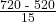= 1.74 The exam score of 720 is 1.74 standard deviations above the mean of 520.
2. z = 1.5
The math SAT score is 520 + 1.5(115) ≈ 692.5. The exam score of 692.5 is 1.5 standard deviations above the mean of 520.
3.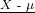=≈ 1.59, the z-score for the SAT.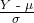=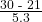≈ 1.70, the z-scores for the ACT. With respect to the test they took, the person who took the ACT did better (has the higher z-score).

<!– <para id=”fs-idp207361600″>Use the following information to answer the next three exercises: X ~ U(3, 13) –>

### Key Terms

Standard Normal Distribution
a continuous random variable (RV) X ~ N(0, 1); when X follows the standard normal distribution, it is often noted as Z ~ N(0, 1).
z-score
the linear transformation of the form z =or written as z =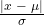; if this transformation is applied to any normal distribution X ~ N(μ, σ) the result is the standard normal distribution Z ~ N(0,1). If this transformation is applied to any specific value x of the RV with mean μ and standard deviation σ, the result is called the z-score of x. The z-score allows us to compare data that are normally distributed but scaled differently. A z-score is the number of standard deviations a particular x is away from its mean value.